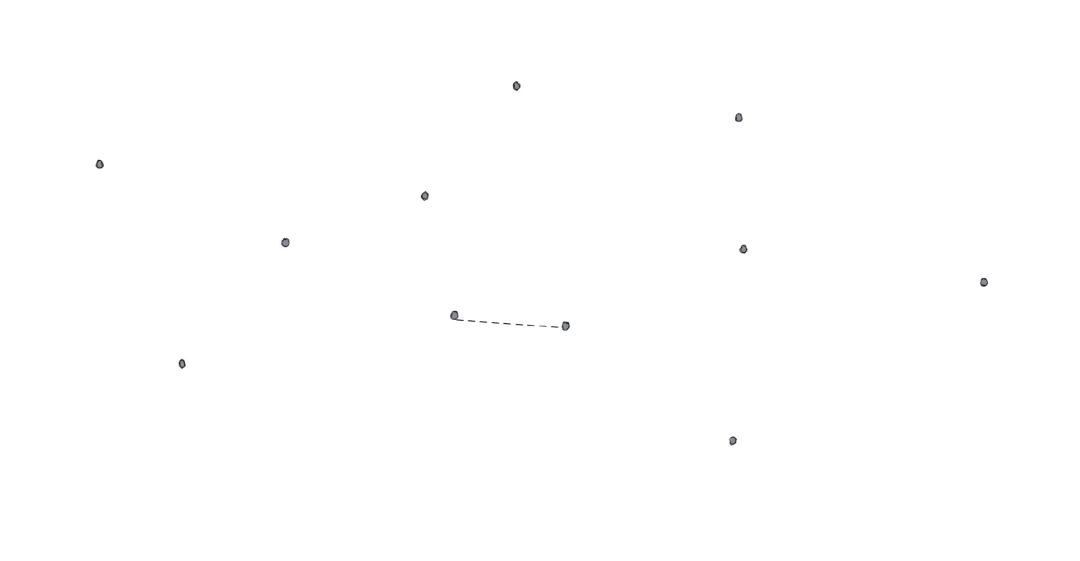• 如果您觉得本站非常有看点，那么赶紧使用Ctrl+D 收藏吧

# 平面内有N个点，如何快速求出距离最近的点对？

2周前 (10-16) 15次浏览

## 题意，因为n个点取两个点一个有

## 分治法

，也就是左右两边最短距离的最小值，这个应该很好理解。

，宽是

，它的对角线长度是

。那么根据鸽笼原理，如果我们放入超过6个点，必然会存在一个小矩形内存在两个点。而小矩形内最大的距离小于D，也就是说这两个点的距离必然也小于D，这就和我们之前的假设矛盾了，所以可以得出超过7个点的情况是不存在的。

## 代码实现

``import randomdef random_point():    x, y = random.uniform(0, 1000), random.uniform(0, 1000)    return (x, y)points = [random_point() for _ in range(200)]``

``def distance(x, y):    return math.sqrt((x - y) ** 2 + (x - y)** 2)def brute_force(points):    ret = sys.maxsize    a, b = None, None    n = len(points)    for i in range(n):        for j in range(i+1, n):            dis = distance(points[i], points[j])            if dis < ret:                ret = dis                a, b = i, j    return ret, points[a], points[b]``

``def divide_algorithm(points):    n = len(points)    # 特判只有一个点或者是两个点的情况    if n < 2:        return sys.maxsize, None, None    elif n == 2:        return distance(points, points), points, points    # 对所有点按照横坐标进行排序    points = sorted(points)    half = (n - 1) // 2    # 递归，这里有一个问题，为什么要先排序再递归？    d1, a1, b1 = divide_algorithm(points[:half])    d2, a2, b2 = divide_algorithm(points[half:])    d, a, b = (d1, a1, b1) if d1 < d2 else (d2, a2, b2)    calibration = points[half]     # 根据中间的位置将点集分成两个部分    left, right = [], []    for u in points:        if calibration - d < u < calibration:            left.append(u)        elif calibration <= u < calibration + d:            right.append(u)    # 右侧点集按照纵坐标排序    right = sorted(right, key=lambda x: (x, x))    res = d    for u in left:        # 左开右闭的二分        l, r = -1, len(right)-1        while r - l > 1:            m = (l + r) >> 1            if right[m] <= u - d:                l = m            else:                r = m        idx = r          # 在范围内最多只有6个点能够构成最近点对        for j in range(7):            if j + idx >= len(right):                break            if distance(u, right[idx + j]) < res:                res = distance(u, right[idx + j])                a, b = u, right[idx + j]    return res, a, b``

– END –